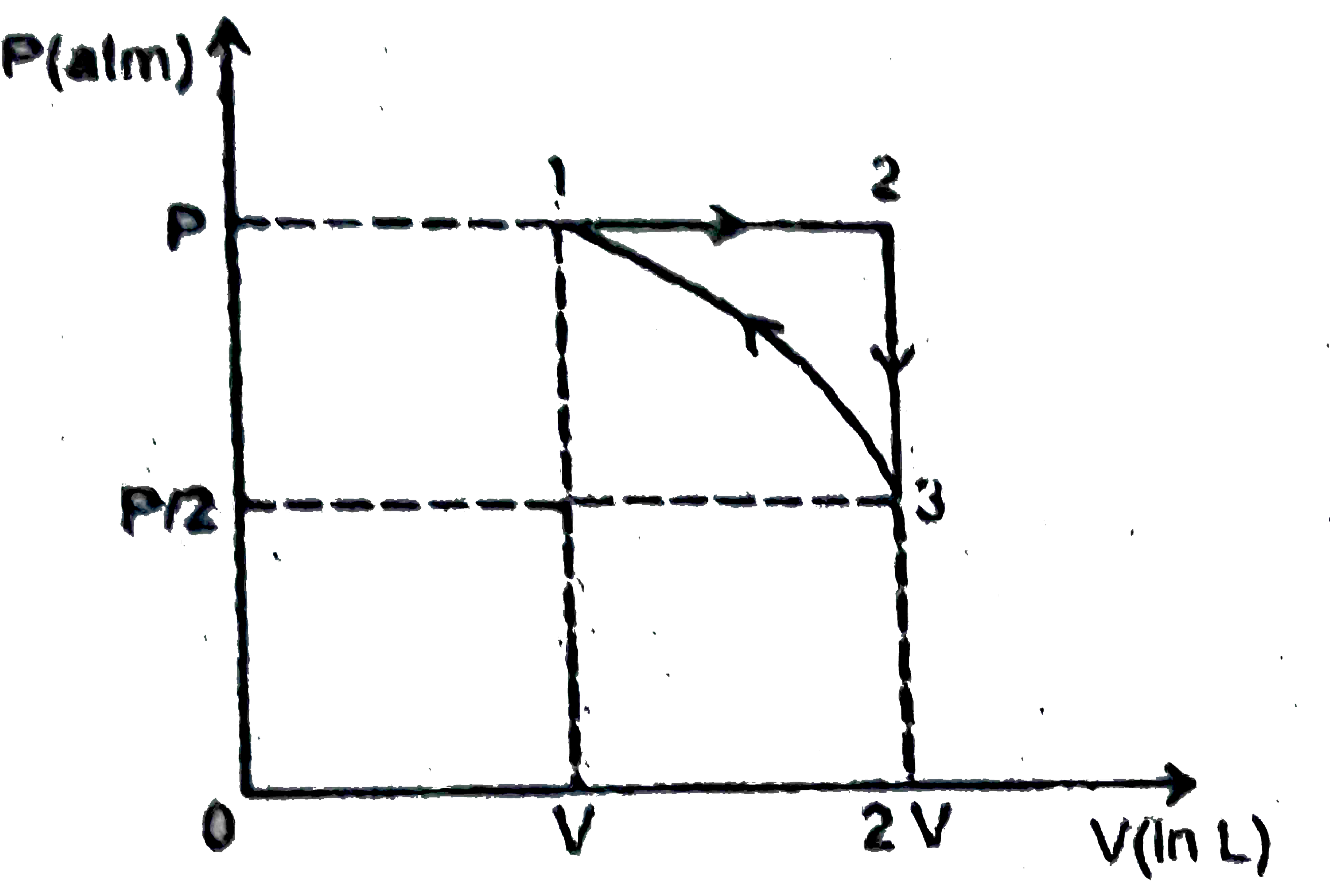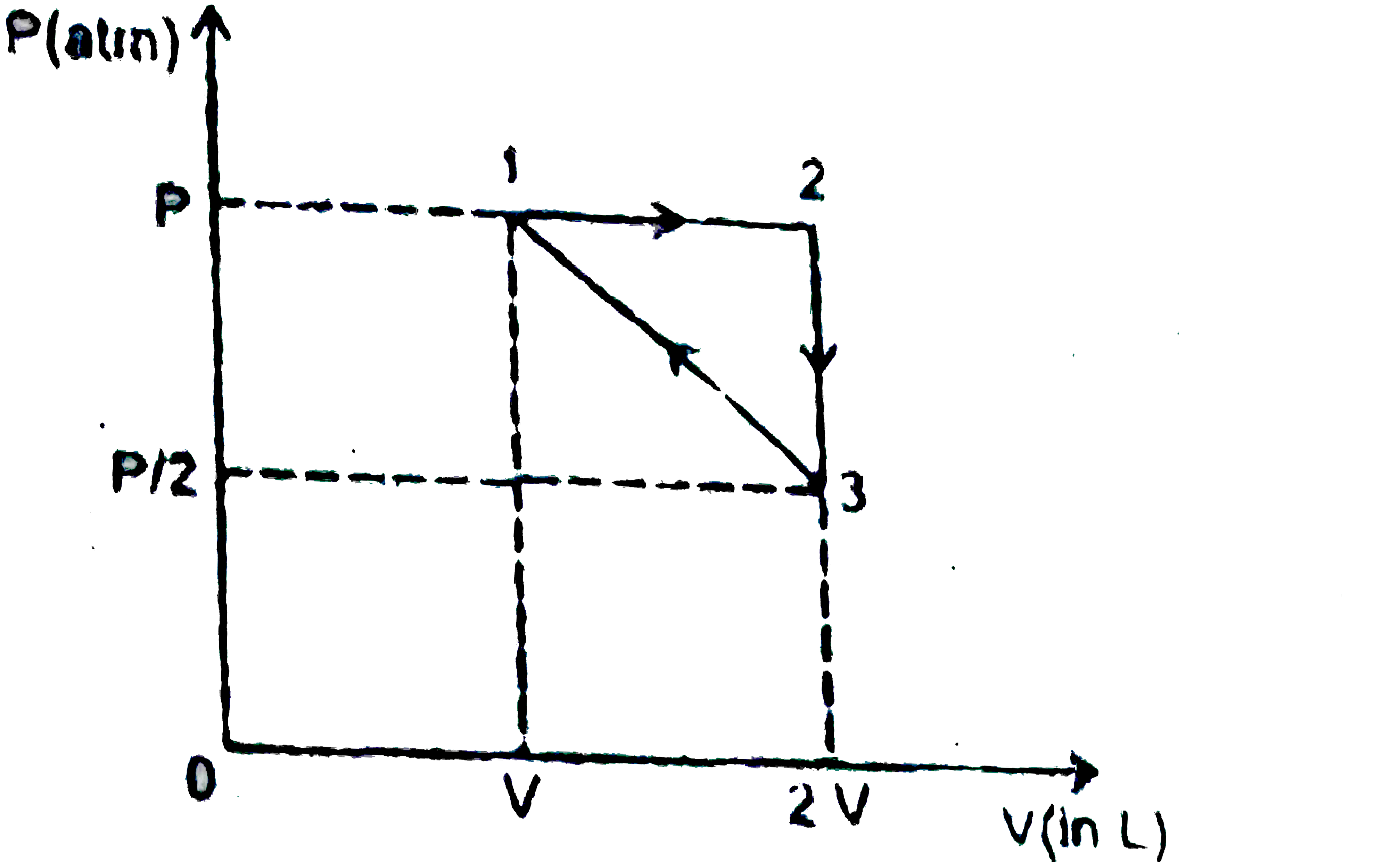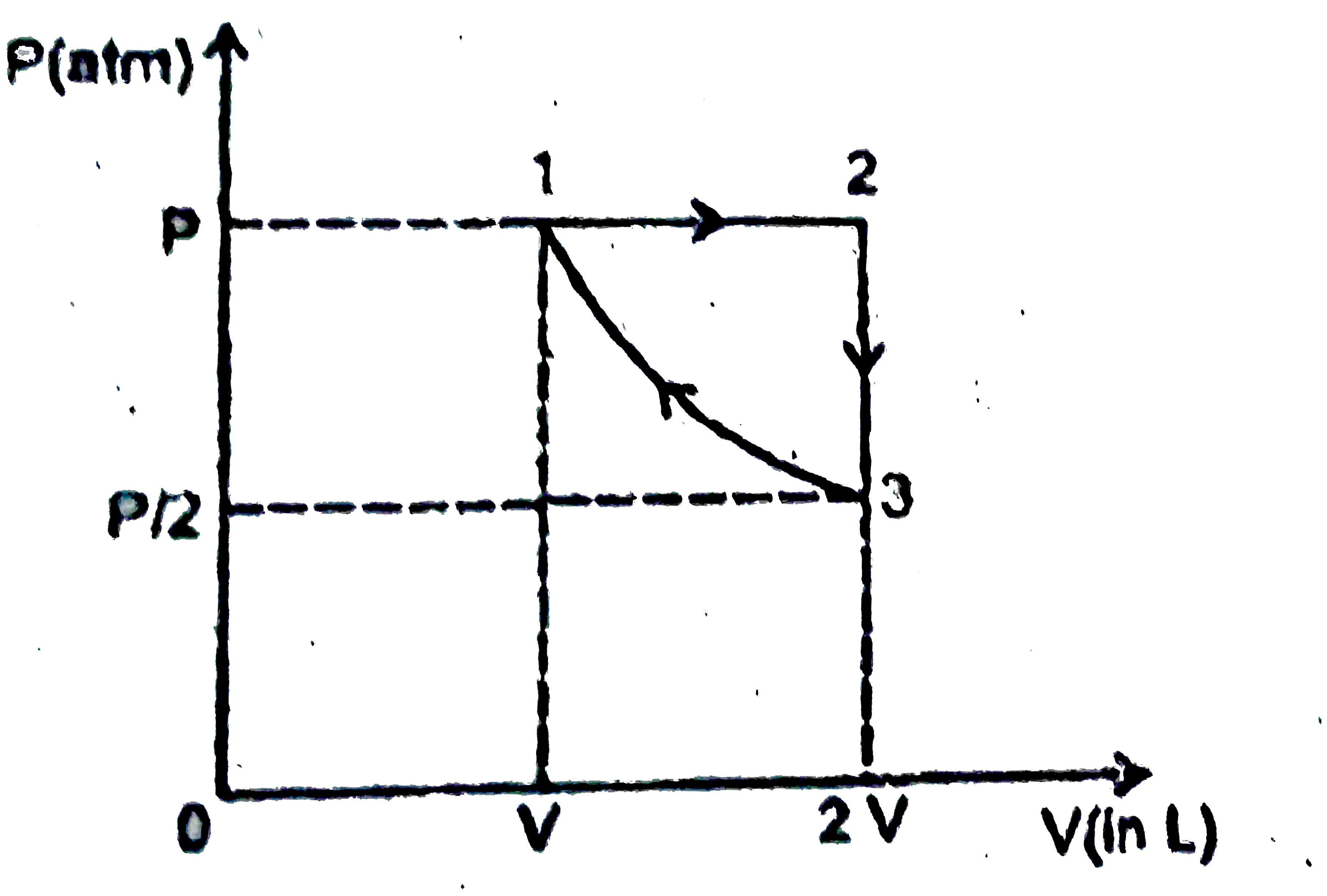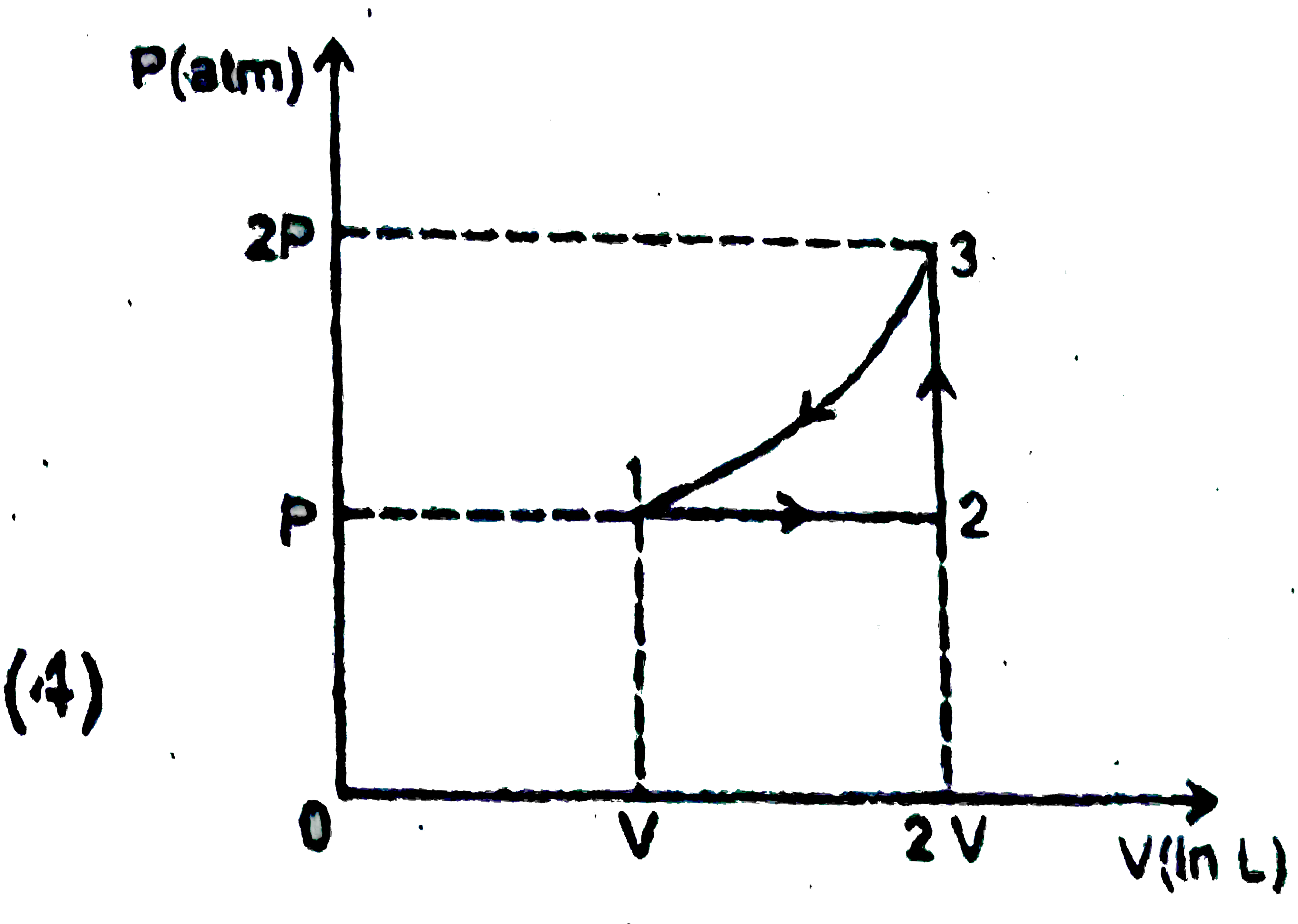# Two moles of an ideal gas undergo the following process : (a) a reversible isobaric expansion from ( P atm, V L) to ( P atm , 2V L). (b)

90 views
Two moles of an ideal gas undergo the following process :
(a) a reversible isobaric expansion from ( P atm, V L) to ( P atm , 2V L).
(b) a reversible isochoric change of state from ( P atm , 2V,L) to (P//2 atm, 2V L)
(c ) a reversible isothermal compression from (P//2 atm, 2V L) to (P  atm, V L).
Sketch with labels each of the processes on the same P-V diagram.
A.B.C.D.by (74.1k points)